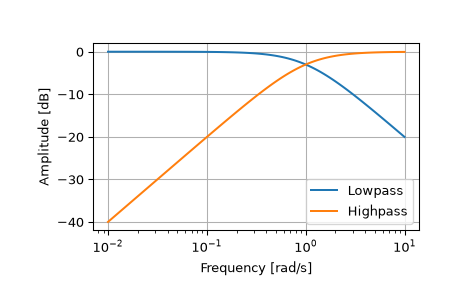# scipy.signal.lp2hp¶

scipy.signal.lp2hp(b, a, wo=1.0)[source]

Transform a lowpass filter prototype to a highpass filter.

Return an analog high-pass filter with cutoff frequency wo from an analog low-pass filter prototype with unity cutoff frequency, in transfer function (‘ba’) representation.

Parameters
barray_like

Numerator polynomial coefficients.

aarray_like

Denominator polynomial coefficients.

wofloat

Desired cutoff, as angular frequency (e.g., rad/s). Defaults to no change.

Returns
barray_like

Numerator polynomial coefficients of the transformed high-pass filter.

aarray_like

Denominator polynomial coefficients of the transformed high-pass filter.

Notes

This is derived from the s-plane substitution

$s \rightarrow \frac{\omega_0}{s}$

This maintains symmetry of the lowpass and highpass responses on a logarithmic scale.

Examples

>>> from scipy import signal
>>> import matplotlib.pyplot as plt

>>> lp = signal.lti([1.0], [1.0, 1.0])
>>> hp = signal.lti(*signal.lp2hp(lp.num, lp.den))
>>> w, mag_lp, p_lp = lp.bode()
>>> w, mag_hp, p_hp = hp.bode(w)

>>> plt.plot(w, mag_lp, label='Lowpass')
>>> plt.plot(w, mag_hp, label='Highpass')
>>> plt.semilogx()
>>> plt.grid()